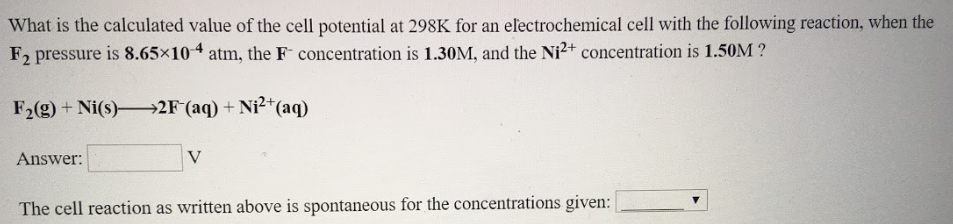# What is the calculated value of the cell potential at 298K for an electrochemical cell with the following reaction, when the F2 pressure is 8.65x10^-4 atm, the F^- concentrations is 1.30M, and the Ni^2+ concentrations is 1.50M? F2(g) + Ni(s) → 2F^-(aq) + Ni^2+(aq) Answer: ____ V The cell reaction as written above is spontaneous for the concentrations given: ____